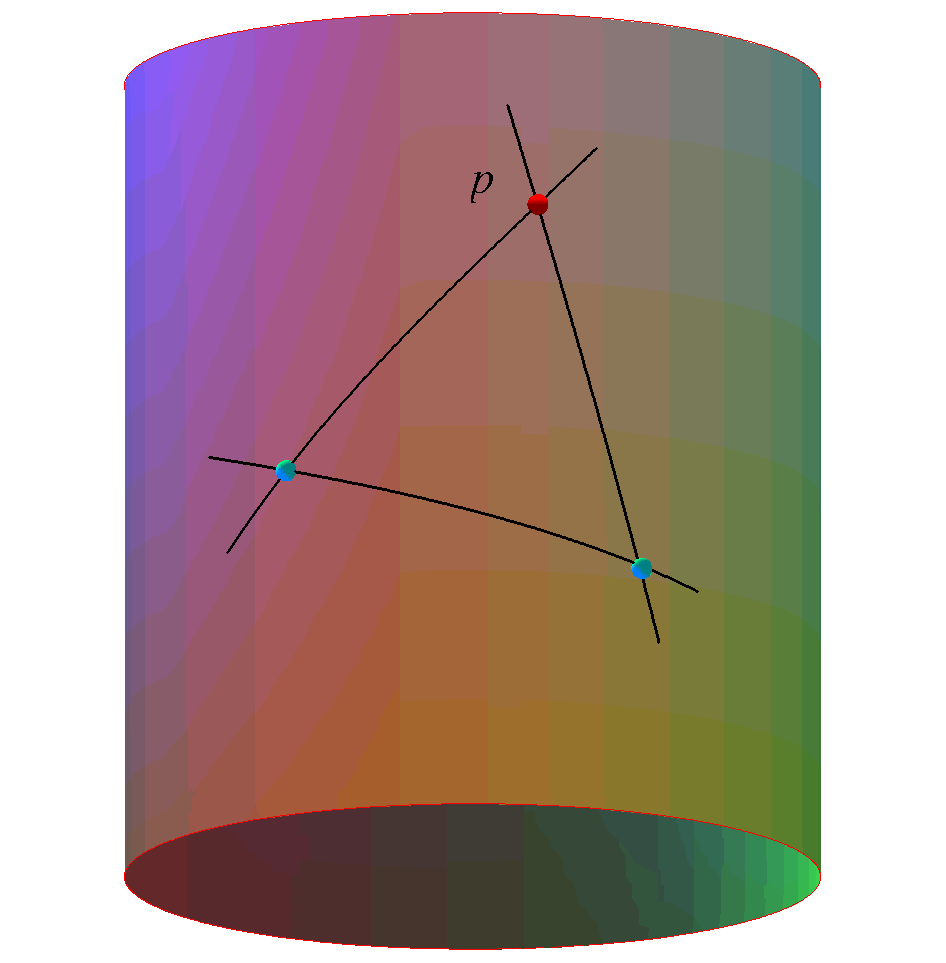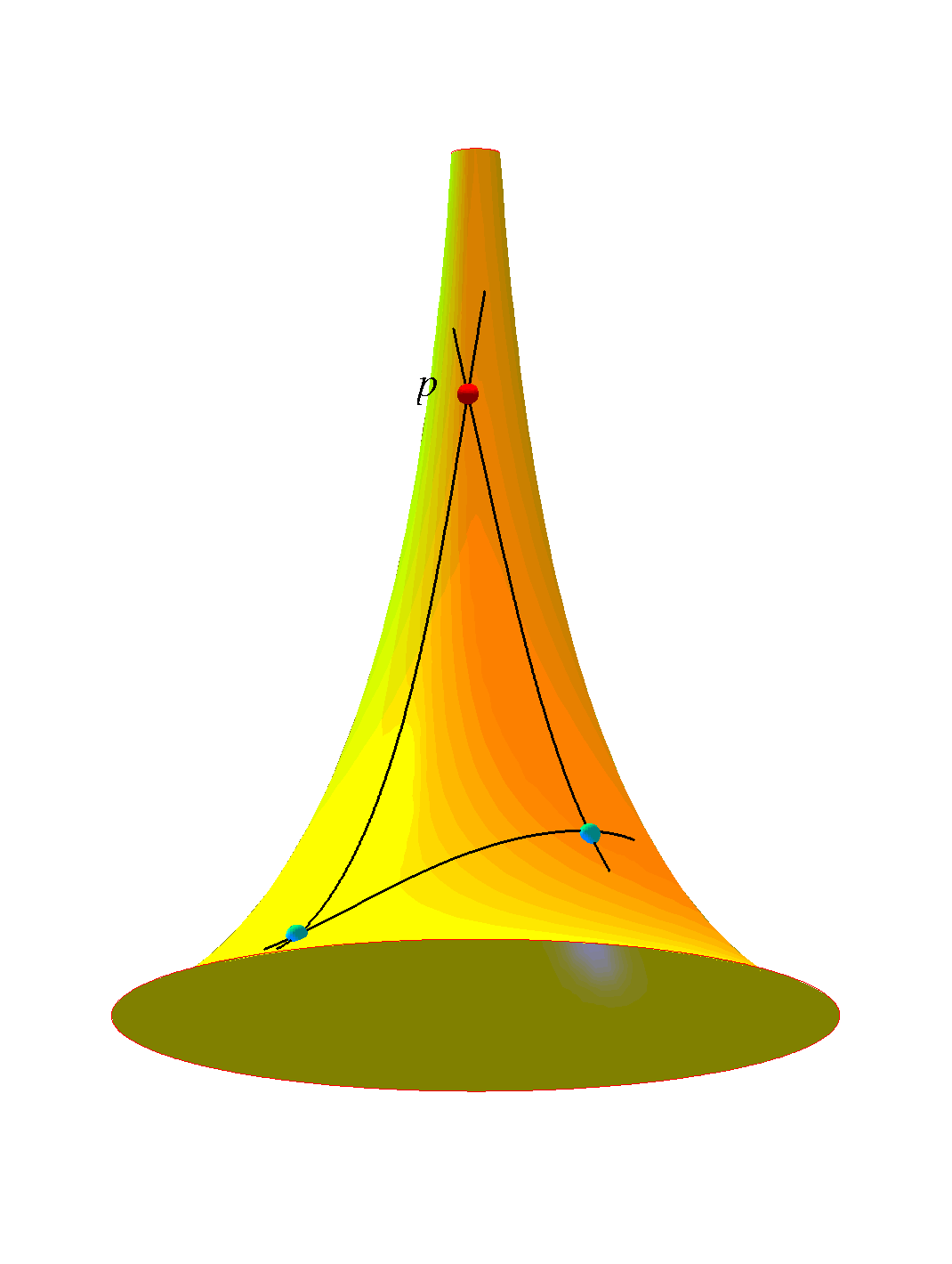# Manifold Learning in Image and Signal Analysis

## Syllabus

From shapes to manifolds
Rasmus R. PaulsenImagine that you have acquired a set of 3D shapes using a medical imaging modality and you would like to use some of the more advanced manifold learning techniques to extract subtle anatomical information from your set of shapes. This talk introduces some of the common methods for transforming sets of shapes into a manifold representation.
The talk will focus on shape representation, correspondence, rigid alignment, ICP, non-rigid registration, and Procrustes analysis.

R. R. Paulsen, R. Larsen, S. Laugesen, C. Nielsen, B. K. Ersbøll, Building and Testing a Statistical Shape Model of the Human Ear Canal, Medical Image Computing and Computer-Assisted Intervention - MICCAI 2002, 5th Int. Conference, Tokyo, Japan, Springer, 2002

Presentation

Principal component analysis and independent component analysis
Ole WintherThis talk will be journey through linear latent variables models starting from principal component analysis (PCA), continuing through the probabilistic generative model-based approach and finally arriving at the Bayesian solution.

Keywords: PCA, singular value decompostion (SVD), probabilistic PCA (pPCA), identifiabillity, Independent component analysis (ICA), Bayesian approach, sparsity.

Presentation

Manifold learning
Mikhail BelkinIn my tutorial I will introduce the basics of manifold learning and its connection to graph-based semi-supervised learning. We will discuss the basics of manifolds and the goals and the applicability of manifold approaches and some of the applications. In particular, we will talk about the Laplace operator on the manifold and how the geometry of data influences the classification boundaries and how unlabeled data can be useful for classification.

S. Roweis, L. Saul. Nonlinear Dimensionality Reduction by Locally Linear Embedding.
JB Tenenbaum, V Silva, JC Langford. A Global Geometric Gramework for Nonlinear Dimensionality Reduction
M. Belkin P. Niyogi. Laplacian Eigenmaps for Dimensionality Reduction and Data Representation
M. Belkin P. Niyogi. Semi-supervised Learning on Riemannian Manifolds

Presentation

Manifolds and Riemannian geometry
Francois Lauze
Presentation

Statistics on manifolds
Thomas FletcherManifold representations are useful for many different types of data, including directional data, transformation matrices, tensors, and shape. Statistical analysis of these data is an important problem in a wide range of image analysis and computer vision applications. However, defining statistics on a manifold is not a straightforward process. Even the simplest statistics, such as the mean, depend on the vector space structure of Euclidean space. This structure is not available for a general manifold. In this lecture I will discuss how many common statistics can be defined for manifold-valued data by utilizing the geodesic distance on the manifold. The first example is the mean value of data on a manifold, which can be defined as the point that minimizes the sum-of-squared geodesic distances to the data. Following this example, we can also define variance, principal components analysis, regression, and the median. Computation of all of these statistics is achieved using only two tools, the Riemannian exponential and log mappings. After explaining the algorithms for computing statistics on manifolds, I will demonstrate their application in several image analysis problems.

P.T. Fletcher, S. Joshi, C. Lu, S.M. Pizer. Principal Geodesic Analysis for the Study of Nonlinear Statistics of Shape, IEEE Transactions on Medical Imaging, vol. 23, no. 8, pp. 995-1005, 2004.
B. Davis, P.T. Fletcher, E. Bullitt, S. Joshi. Population shape regression from random design data, IEEE International Conference on Computer Vision (ICCV), October, 2007.
P.T. Fletcher, S. Venkatasubramanian, S. Joshi. The Geometric Median on Riemannian Manifolds with Application to Robust Atlas Estimation, In Neuroimage, vol. 45, pp. S143-52, 2009.

Presentation

Scientific visualization with manifolds
Lars Kai Hansen
Unsupervised learning, independent components, kernel methods, and the pre-image problem. Neuroimaging, social networks, and audio signalprocessing.

Presentation

The meaning of curvature - A distance geometric approach
Steen MarkvorsenThis talk is essentially concerned with the shape molding forces of curvature and with the old question of how to detect the presence of curvature in manifolds and length spaces at various scales ranging from global to local to microlocal.
As the old saying goes: Positive curvatures produce fat (geodesic) triangles and negative curvatures slim triangles.
Some basic ideas from metric (length-)space geometry and from the geometric analysis of the Laplacian will be surveyed and applied.
Via the Laplacian the curvature tensor is in control of a variety of very natural phenomena ranging from heat diffusion to volume growth. We are e.g. interested in obtaining precise bounds for mean exit times for Brownian motions and for isoperimetric inequalities.
One way to obtain such bounds are via curvature controlled comparison with corresponding values in constant curvature spaces and in other tailor-made so-called warped product spaces.

Ana Hurtado, S. Markvorsen, and Vicente Palmer. Torsional Rigidity of Submanifolds with controlled Geometry. Mathematische Annalen 344 (2009) 511 - 542.
S. Markvorsen and V. Palmer. Extrinsic Isoperimetric Analysis on Submanifolds with Curvatures bounded from below. Accepted for publication in Journal of Geometric Analysis (2009).
S.Markvorsen. Minimal Webs in Riemannian Manifolds. Geometriae Dedicata 133 (2008) 7 - 34
Presentation

Sparse statistical models for relating anatomical differences to clinical outcome
Rasmus LarsenRecent advances in statistics have spawned powerful methods for regression and data decomposition that promote sparsity, a property that facilitates interpretation of the results. Sparse models use a small subset of the available variables and may perform as well or better than their full counterparts if constructed carefully.

Karl Sjöstrand et al. Sparse Decomposition and Modeling of Anatomical Shape Variation. IEEE Transactions on Medical Imaging. vol: 26, issue: 12, pages: 1625-1635, 2007
H. Zou and T. Hastie. Regularization and variable selection via the elastic net. Journal of the Royal Statistical Society: Series B (Statistical Methodology), 67(2):301-320, 2005.

Presentation

Human Motion Analysis and Manifolds
Kim Steenstrup PedersenI will in this lecture give an overview of human motion analysis and how manifold representations naturally arise as representations of the constrained articulated motion performed by humans.

R. Poppe. Vision-based human motion analysis: An overview. Computer Vision and Image Understanding, 108 (1-2): 4-18, 2007.

Presentation

Estimating covariance matrices
Jon Sporring

Presentation

Applications of shape manifolds in medical image analysis.